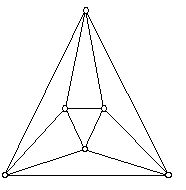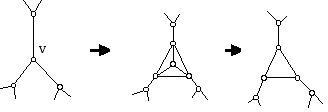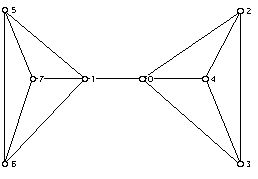Joseph Malkevitch: Research Frontier: Discrete Geometry

The Research Frontier

prepared by:

Joseph Malkevitch
Department of Mathematics and Computing
York College (CUNY)
Jamaica, New York 11451

Email: malkevitch@york.cuny.edu

In many situations involving discrete geometry questions, extensions of exercises lead to questions whose answers may not be known. Not all of such problems are either "interesting" or "important." However, it is good practice to learn how to generate problems of this kind. It is also fun to investigate such questions and sometimes they are important. One never knows when the methods that one develops to answer a question of limited interest might be the right tool to answer some very important question. The questions below are to encourage you to think about questions which can be made to probe the research frontier.

1. The diagram below (Figure 1) shows an example of a plane 4-valent (simple) graph.Figure 1

This diagram can be interpreted as a projection of a knot in the sense that if one is moving along an edge and one reaches a vertex, there is an edge to the left, one in the middle, and one on the right. Suppose one always takes the middle choice. Figure 2 shows a cut-through pattern at vertex v.Figure 2

For the graph in Figure 1, moving along any edge in this way creates an Eulerian circuit. For 4-valent graphs such an Eulerian circuit will be called "cut-through" Eulerian. Thus, we can interpret this graph as having arisen by projecting a knot into the plane.

a. Write down the degree sequence of this graph.

b. Can you find a graph with the same degree sequence as this graph which is not isomorphic to it?

c. Can you find a plane graph with the same degree sequence as this graph which is not isomorphic to it?

What degree sequences have the property that they can be realized by unique plane graphs (up to isomorphism)?

d. Write down the face vector of the graph in Figure 1.

e. Is there a plane graph with p3 = 8 and p4 = k (k =0, k at least 2)?

f. Is there a plane graph with p3 = 8 and p4 = k (k =0, k at least 2) which is
the projection of a knot?

g. What is the largest size face that can occur in a plane 3-connected 4-valent graph with 2n (n at least 3) vertices?

h. What is the largest sized face that can occur in a plane 3-connected 4-valent graph with 2n - 1 (n at least 5) vertices?

Which face vectors arise as projections of a knot if digons and loops are not allowed?

2. The two extremes for "cut-through paths" in 4-valent plane (especially 3-connected) graphs G are:

a. Cut-through Eulerian

b. G is the union of simple closed curves

Figure 1 provides an example of a. and the graph of the (regular) octahedron (Figure 3) provides an example of b.Figure 3

It is often possible to perform various "operations" on plane (3-connected) graphs which preserve "interesting" properties of the graphs. For example, in the part of a 4-valent plane graph shown in Figure 4, one can take any vertex and create a "circuit" of vertices around it. This operation will preserve being plane 4-valent and 3-connected, and if the original graph is the union of simple closed cut-through circuits so will the new graph. However, if the original graph was cut-through Eulerian, this property would no longer hold.Figure 4

Find operations which preserve being cut-through Eulerian for plane 4-valent 3-connected graphs and which are related to the start graph in ways that are "interesting."

The operation shown in Figure 4 is related to an important geometrical operation on polyhedra, known as truncation. When one truncates a vertex of a (convex) polyhedron one cuts the vertex off with a plane. Thus, a vertex which was i-valent gets replaced by some new 3-valent vertices and a new face which has i sides. In graph theory terms this can be thought of as a two- stage operation where one puts a circuit with i edges around an i-valent vertex v, and then erases the original vertex v and edges present at v, as shown in Figure 5. In the graph theory setting one can think of both of the resulting graphs to the right of the original one as interesting "new" graphs. In the geometrical case, the result of the truncation is shown at the far right, but the middle diagram can be thought of as truncating the vertex v and then erecting a "pyramid" on the face of the polyhedron that was created when v was cut off. A large variety of interesting questions can be posed related to performing these operations on all of the vertices of a graph or some of the vertices of a graph, or obtaining a family of graphs by performing a sequence of such operations, starting from some "interesting" graph.Figure 5

3. Look for sufficient conditions for a plane 4-valent (3-connected) graph to decompose into cut-through simple closed curves. The simplest example of such a graph is the one shown in Figure 2. Necessary conditions for this to happen are also of interest.

4. A (simple) graph is said to be hamiltonian (in honor of the Irish mathematician William Rowan Hamilton) if there is a circuit which passes through all of its vertices. A circuit of this type will be called a hamiltonian circuit.

a. Does Figure 2 have a hamiltonian circuit? Does it have a hamiltonian circuit so that the edges not in the hamiltonian circuit (that is, the complementary edges) also form a hamiltonian circuit? Find examples of plane 4-valent 3-connected graphs which have a hamiltonian circuit but do not admit edge disjoint hamiltonian circuits. Infinite families of such graphs would be of especial interest.

b. Does the truncation operation on a single vertex of a graph always preserve the property of having a hamiltonian circuit for a graph ? Does the truncation operation on a single vertex always preserve the property of not having a hamiltonian circuit for a graph ? For what graphs will it preserve the property of being hamiltonian? For what graphs will it preserve the property of being non-hamiltonian?

c. Are there graphs, which when one simultaneously truncates all of the vertices of the graph, one gets a new graph which has a hamiltonian circuit?

d. How does the truncation operation interact with the property of a graph having an Eulerian circuit or an (open) Eulerian path?

Note:

The particular interest in studying planar 3-connected graphs is that a theorem of Ernst Steinitz, as reformulated in graph theory terms by Branko Grünbaum and Theodore Motzkin, shows that such graphs are isomorphic to the vertex-edge graphs of 3-dimensional convex (bounded) polyhedra.

5. Among the many interesting ways to transform a (simple) graph into another graph are the line graph and the medial graph. The line graph L(G) of a graph G is obtained as follows. Each vertex of L(G) corresponds to an edge of G. Join two vertices of L(G) if the edges they correspond to in G share a vertex. Figure 6 shows an example, where the line graph has 9 vertices because G, on the left, has 9 edges.Figure 6

a. Can you label the vertices of the graph on the right in Figure 6 with the pairs of vertex labels of the graph on the left which represent the edges of the original graph?

b. Is the graph on the right in Figure 6 a planar graph?

c. Can you find conditions on a graph such that its line graph will be planar?

The medial graph M(G) of a plane graph G is obtained as follows. M(G) has a vertex for each edge of G (just as the line graph does). Join two vertices of M(G) if the edges they represent have a vertex in common as well as lie in the same face. Note that the medial graph of a (simple) graph may turn out to have multiple edges.
d. Draw the medial "graph" of the graph on the left in Figure 6.

e. Draw the medial graph of the graph in Figure 3.

f. Draw the medial graph of the graph in Figure 1.

g. When are the medial and line graphs of a graph isomorphic?

h. Draw the medial and line graphs for the graph of the tetrahedron (Figure 7). (Note: Figure 7 shows two different drawings of the tetrahedron graph in the plane. The one on the left is plane and the one on the right is not. However, the one on the left requires 6 different slopes to draw the graph in the plane while the graph on the left requires only 4 different slopes.)Figure 7

i. Draw the medial graph of the graph in Figure 3. Do you recognize the graph you get as in some way familiar?

j. Can you find simple conditions on the plane (simple) graph H such that the medial graph of H is 4-valent and plane.

k. What is the medial graph of the graph shown in Figure 8?Figure 8

5. The regular tetrahedron T (all its faces are congruent equilateral triangles) as a convex polyhedron has a symmetry group. (By symmetry group, here, I will mean the group of isometries (distance preserving transformations) of the object onto itself.) For now, distances will refer to Euclidean distance. As Figure 7 shows, one can represent T in the plane by graphs which have different looking drawings. What is the symmetry group of the most symmetrical "version" of the graph on the left in Figure 7 as a graph in the plane? What is the symmetry group of the most symmetrical "version" of the graph drawn on the right?

If G is a graph, one can define the automorphism group of G. One labels the vertices of G and looks at the group of 1:1 mappings of the vertices onto themselves that preserve the edges between the vertices of the graph.

What is the automorphism group of the graph of the tetrahedron?

6. Figure 9 shows a drawing of the graph of a cube in the plane.Figure 9

Can you find other interesting drawings of the cube in the plane?